Home Practice
For learners and parents For teachers and schools
Textbooks
Full catalogue
Pricing SupportLog in

We think you are located in United States. Is this correct?

# Ordering, comparing and place value of decimal fractions

## 14.2 Ordering, comparing and place value of decimal fractions

### Decimal fraction notation

Decimal fractions and common fractions are simply different ways of expressing the same number. We call them different notations.

decimal fraction
a decimal fraction is a fraction in which the digits of the numerator are written after a decimal point

To write a common fraction as a decimal fraction, we must first express the common fraction using a power of ten ($$10$$, $$100$$, $$1\ 000$$, etc.) as the denominator. For example:

\begin{align} \frac{9}{20} &= \frac{9}{20} \times \frac{5}{5} \\ &= \frac{45}{100} \\ &= \text{0,45} \end{align}

You can also use a calculator to divide the numerator by the denominator to get the decimal form of a fraction, for example:

\begin{align} \frac{9}{20} &= 9 \div 20 \\ &= \text{0,45} \end{align}

To write a decimal fraction as a common fraction, we must first express it as a common fraction with a power of ten as denominator, and then simplify the fraction if necessary. For example:

\begin{align} \text{0,65} &= \frac{65}{100} \\ &= \frac{65 \div 5}{100 \div 5} \\ &= \frac{13}{20} \end{align}

### Place value

Each digit in the number has its own place value. For example, in the number $$\text{1,23}$$, the digit “$$3$$” is in the second decimal place. The second place is called the “hundredths place”.

place value
the value of a digit according to its position in a number

For decimal fractions, the place values we work with are $$\frac{1}{10}$$, $$\frac{1}{100}$$, $$\frac{1}{1\ 000}$$ and $$\frac{1}{10\ 000}$$. They are called tenths, hundredths, thousandths and ten thousandths.

These place values are represented from biggest to smallest, moving to the right after the decimal comma:

units (U) , tenths (t) hundredths (h) thousandths (th) ten thousandths (tth)
$$1$$ , $$\frac{1}{10}$$ $$\frac{1}{100}$$ $$\frac{1}{1\ 000}$$ $$\frac{1}{10\ 000}$$

The fractions for these place values are written in this way:

• $$\text{0,1}$$ means zero units and one $$\frac{1}{10}$$
• $$\text{0,01}$$ means zero units, zero tenths and one $$\frac{1}{100}$$
• $$\text{0,001}$$ means zero units, zero tenths, zero hundredths and one $$\frac{1}{1\ 000}$$
• $$\text{0,0001}$$ means zero units, zero tenths, zero hundredths, zero thousandths and one $$\frac{1}{10\ 000}$$.

In the same way as for whole numbers, we move to a new place value each time we reach $$10$$. For example, this block of squares is divided into $$100$$ parts.

One hundredth, which is $$\frac{1}{100} = \text{0,01}$$, may be shown like this: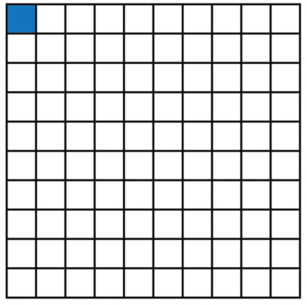Four parts of this block of $$100$$ squares, which is $$4 \times \frac{1}{100} = \frac{4}{100} = \text{0,04}$$, may be shown like this: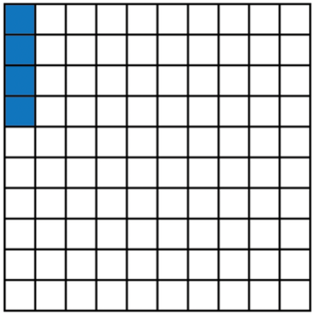## Worked Example 14.1: Finding place value in decimal numbers

Write the place values of all the non-zero digits in the following number: $$\text{73 025,048}$$

### Write the number in a place value table. Show the decimal point clearly.

TTh
$$(\text{10 000})$$
Th
$$(\text{1 000})$$
H
$$(\text{100})$$
T
$$(\text{10})$$
U
$$(\text{1})$$
, t
$$(\text{0,1})$$
h
$$(\text{0,01})$$
th
$$(\text{0,001})$$
tth
$$(\text{0,0001})$$
$$7$$ $$3$$ $$0$$ $$2$$ $$5$$ , $$0$$ $$4$$ $$8$$

### Multiply each non-zero digit by the value of the column it is in.

• $$7 \times 10\ 000 = 70\ 000$$
• $$3 \times 1\ 000 = 3\ 000$$
• $$2 \times 10 = 20$$
• $$5 \times 1 = 5$$
• $$4 \times \text{0,01} = \text{0,04}$$
• $$8 \times \text{0,001} = \text{0,008}$$

As you can see, we do not give the place value of the zeroes in a number, because $$0$$ multiplied by anything equals $$0$$. So, the place value of any $$0$$ will always be $$0$$.

The zeroes have to be included in a number to show the place values of the other digits. This is why we call $$0$$ a placeholder.

When comparing decimal numbers, you can place them on the same place value table and compare the digits from left to right. For example, to compare $$\text{36 601,25}$$ with $$\text{36 705,75}$$ we can place these numbers in the table and compare the digits:

TTh
($$\text{10 000}$$)
Th
($$\text{1 000}$$)
H
($$\text{100}$$)
T
($$\text{10}$$)
U
($$\text{1}$$)
, t
($$\text{0,1}$$)
h
($$\text{0,01}$$)
th
($$\text{0,001}$$)
tth
($$\text{0,0001}$$)
3 6 6 0 1 , 2 5
3 6 7 0 5 , 7 5

The hundred digits are different: $$6 < 7$$. So the first decimal number is smaller than the second: $$\text{36 601,25} < \text{36 705,75}$$. We do not need to compare the digits that follow.

The number with fewer digits before the decimal comma is always smaller, so $$\text{999,99} < \text{1 111,11}$$.

Exercise 14.1

Write down the place value of the given digit in each case.

1. Give the values of the digits $$5$$ and $$8$$ in the number $$\text{5 021,08}$$.
2. Give the values of the digits $$1$$ and $$4$$ in the number $$\text{75,1804}$$.
3. Give the values of the digits $$9$$ and $$7$$ in the number $$\text{10 591,073}$$.
4. Give the two values of the digit $$5$$ in the number $$\text{30 502,51}$$.
5. Give the three values of the digit $$9$$ in the number $$\text{99 178,95}$$.
1. The digits $$5$$ and $$8$$ in the number $$\text{5 021,08}$$:

TTh
($$\text{10 000}$$)
Th
($$\text{1 000}$$)
H
($$\text{100}$$)
T
($$\text{10}$$)
U
($$\text{1}$$)
, t
($$\text{0,1}$$)
h
($$\text{0,01}$$)
th
($$\text{0,001}$$)
tth
($$\text{0,0001}$$)
5 0 2 1 , 0 8
• $$5 \times 1\ 000 = \text{5 000}$$
• $$8 \times \text{0,01} = \text{0,08}$$
2. The digits $$1$$ and $$4$$ in the number $$\text{75,1804}$$:

TTh
($$\text{10 000}$$)
Th
($$\text{1 000}$$)
H
($$\text{100}$$)
T
($$\text{10}$$)
U
($$\text{1}$$)
, t
($$\text{0,1}$$)
h
($$\text{0,01}$$)
th
($$\text{0,001}$$)
tth
($$\text{0,0001}$$)
7 5 , 1 8 0 4
• $$1 \times \text{0,1} = \text{0,1}$$
• $$4 \times \text{0,0001} = \text{0,0004}$$
3. The digits $$9$$ and $$7$$ in the number $$\text{10 591,073}$$:

TTh
($$\text{10 000}$$)
Th
($$\text{1 000}$$)
H
($$\text{100}$$)
T
($$\text{10}$$)
U
($$\text{1}$$)
, t
($$\text{0,1}$$)
h
($$\text{0,01}$$)
th
($$\text{0,001}$$)
tth
($$\text{0,0001}$$)
1 0 5 9 1 , 0 7 3
• $$9 \times 10 = 90$$
• $$7 \times \text{0,01} = \text{0,07}$$
4. The two values of the digit $$5$$ in the number $$\text{30 502,51}$$:

TTh
($$\text{10 000}$$)
Th
($$\text{1 000}$$)
H
($$\text{100}$$)
T
($$\text{10}$$)
U
($$\text{1}$$)
, t
($$\text{0,1}$$)
h
($$\text{0,01}$$)
th
($$\text{0,001}$$)
tth
($$\text{0,0001}$$)
3 0 5 0 2 , 5 1
• $$5 \times 100 = 500$$
• $$5 \times \text{0,1} = \text{0,5}$$
5. The three values of the digit $$9$$ in the number $$\text{99 178,95}$$:

TTh
($$\text{10 000}$$)
Th
($$\text{1 000}$$)
H
($$\text{100}$$)
T
($$\text{10}$$)
U
($$\text{1}$$)
, t
($$\text{0,1}$$)
h
($$\text{0,01}$$)
th
($$\text{0,001}$$)
tth
($$\text{0,0001}$$)
9 9 1 7 8 , 9 5
• $$9 \times 10\ 000 = 90\ 000$$
• $$9 \times 1\ 000 = 9\ 000$$
• $$9 \times \text{0,1} = \text{0,9}$$

Compare the following decimal numbers.

1. $$\text{77 601,5}$$ and $$\text{77 599,9}$$
2. $$\text{98 713,41}$$ and $$\text{99 100,99}$$
3. $$\text{0,543}$$ and $$\text{10,599}$$
4. $$\text{32 100,51}$$ and $$\text{32 100,59}$$
1. $$\text{77 601,5}$$ and $$\text{77 599,9}$$
TTh
($$\text{10 000}$$)
Th
($$\text{1 000}$$)
H
($$\text{100}$$)
T
($$\text{10}$$)
U
($$\text{1}$$)
, t
($$\text{0,1}$$)
h
($$\text{0,01}$$)
th
($$\text{0,001}$$)
tth
($$\text{0,0001}$$)
7 7 6 0 1 , 5
7 7 5 9 9 , 9

In the hundreds place, $$6 > 5$$, so $$\text{77 601,5} > \text{77 599,9}$$.

2. $$\text{98 713,41}$$ and $$\text{99 100,99}$$
TTh
($$\text{10 000}$$)
Th
($$\text{1 000}$$)
H
($$\text{100}$$)
T
($$\text{10}$$)
U
($$\text{1}$$)
, t
($$\text{0,1}$$)
h
($$\text{0,01}$$)
th
($$\text{0,001}$$)
tth
($$\text{0,0001}$$)
9 8 7 1 3 , 4 1
9 9 1 0 0 , 9 9

In the thousands place $$8 < 9$$, so $$\text{98 713,41} < \text{99 100,99}$$.

3. $$\text{0,543}$$ and $$\text{10,599}$$
TTh
($$\text{10 000}$$)
Th
($$\text{1 000}$$)
H
($$\text{100}$$)
T
($$\text{10}$$)
U
($$\text{1}$$)
, t
($$\text{0,1}$$)
h
($$\text{0,01}$$)
th
($$\text{0,001}$$)
tth
($$\text{0,0001}$$)
0 , 5 4 3
1 0 , 5 9 9

$$\text{0,543}$$ has only one digit before the comma, so $$\text{0,543} < \text{10,599}$$

4. $$\text{32 100,51}$$ and $$\text{32 100,59}$$
TTh
($$\text{10 000}$$)
Th
($$\text{1 000}$$)
H
($$\text{100}$$)
T
($$\text{10}$$)
U
($$\text{1}$$)
, t
($$\text{0,1}$$)
h
($$\text{0,01}$$)
th
($$\text{0,001}$$)
tth
($$\text{0,0001}$$)
3 2 1 0 0 , 5 1
3 2 1 0 0 , 5 9

In the hundredths place $$1 < 9$$, so $$\text{32 100,51} < \text{32 100,59}$$.

### Number lines

Decimal numbers work by dividing things into tens. The vertical indicators on the number line in the diagram below divide the space from zero to one into $$10$$ parts. These indicators represent the numbers $$\text{0,1}$$ and then $$\text{0,2}$$ and $$\text{0,3}$$, and so on until you get to $$1$$.

The scale on the number line is $$\text{0,2}$$, so some numbers are skipped. For example, $$\text{0,3}$$ is at the indicator between $$\text{0,2}$$ and $$\text{0,4}$$.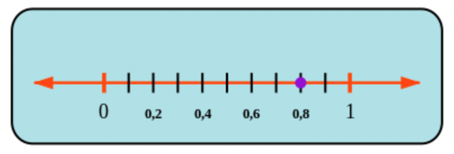The dot is on the number $$\text{0,8}$$.

You can also think of this number as a fraction, which is $$\frac{8}{10}$$ because the picture shows $$8$$ parts out of $$10$$.

The number line is useful for comparing decimal numbers. For example, the diagram below shows the position of $$\text{0,5}$$ (a half) on the number line. You can see that $$\text{0,8}$$ is more (bigger) than $$\text{0,5}$$. We can write this as an inequality: $$\text{0,8} > \text{0,5}$$.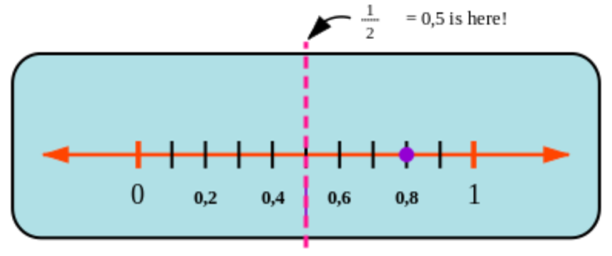When there are more decimals to compare, placing them in order on the number line helps you to find the answer:

There are four numbers marked with dots on the number line below. The points are labelled $$A$$, $$B$$, $$C$$ and $$D$$.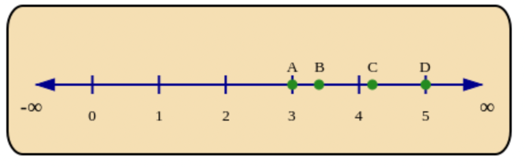You can easily see that $$A < B < C < D$$.

Now if you were given decimal numbers: $$\text{3,4}; \text{5,0}; \text{4,2}; \text{3,0}$$, you could check their position on the number line and place them in order from smallest to biggest.

\begin{align} A &= \text{3,0} \\ B &= \text{3,4} \\ C &= \text{4,2} \\ D &= \text{5,0} \end{align}

If we extend the number line in the negative direction, then we can also compare negative decimal numbers.

Remember, a positive number is always greater than a negative number, and zero is in between the positives and negatives! For example, $$- \text{0,8} < \text{0,39}$$.

If both numbers are negative, then the number further to the left on the number line is smaller. For example, $$- \text{0,8} < - \text{0,39}$$.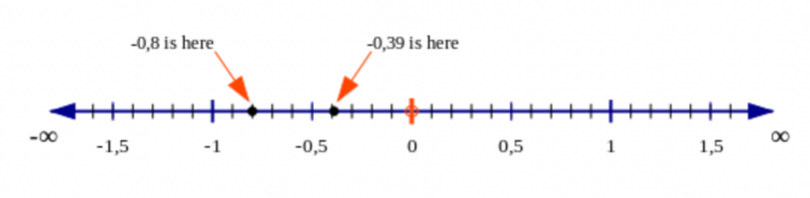temp text